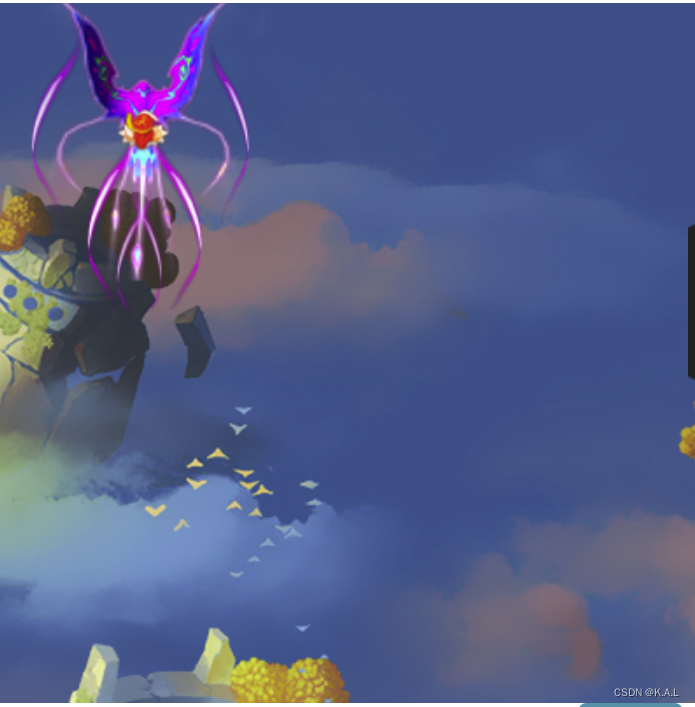# 模块1 制作游戏界面  （基础）

### 1.创建应用

``````var app=new PIXI.Application(512,768);  //用于创建游戏窗口，宽度512像素，高度768像素
//var app表示定义了一个应用程序对象，叫做app
document.body.appendChild(app.view);   //将应用显示到浏览器姐界面``````

### 2.创建图片

``````var  bg=new PIXI.Sprite.fromImage("图片内容来源地址"); //创建一个图片
//app.stage 表示应用程序舞台（所有需要显示的元素，必须放到舞台上）``````

### 3.创建文本

``````var score=new PIXI.Text("得分:000000");  //创建一个文本
score.style.fill="0xffffff";  //设置文本字体颜色
score.x=310;  //名字为score的文本的水平位置为310像素的位置
score.y=10;   //名字为score的文本的水平位置为310像素的位置``````

### 元素常用属性

 属性 属性名称 作用 公共属性 x x坐标 公共属性 y y坐标 公共属性 width 元素宽度 公共属性 height 元素高度 公共属性 rotation 旋转弧度 公共属性 scale 缩放比例 公共属性 visible 是否可见 公共属性 alpha 透明度 文本属性 text 显示内容 文本属性 style 显示 样式

# 模块2 添加游戏控制（鼠标控制）

### 1.鼠标控制事件

 事件 作用 click 点击某个显示元素 mousemove 鼠标在某个显示元素中移动 mousedown 在某个元素上按下鼠标 mouseup 在某个元素上松开鼠标 mouseover 鼠标被移动到某个显示元素上 mouseover 鼠标从某个显示元素上离开

### 2.触屏控制事件

 事件 作用 touchstart 手指触碰屏幕 touchend 手指离开屏幕 touchmove 手指在屏幕上滑动

``````bg.interactive=true;  //允许图片接收控制
bg.on("touchstart",moveCar) ;  //bg添加touchstart事件
bg.on("click",moveCar);        //bg添加click事件
//定义moveCar函数，控制小车移动
function  moveCar(){
car.y-=10;
}
#function定义一个函数``````

### 3.获得鼠标坐标

``````//event  代表当前鼠标的控制事件，该事件中存储了鼠标的相关信息
var pos=event.data.getLocalPosition(app.stage);  //获得鼠标信息，并存储到post变量中
var x=pos.x    //通过post获得鼠标指针的x坐标
var y=pos.y    //通过post获得鼠标指针的x坐标
console.log(..) //在浏览器控制台输出相关信息``````

### 4.鼠标跟随

``````bg.interactive=true;
bg.on("mousemove",movePlane);
function move1{
var pos=event.data.getLocalPostion(app.stage);
plane.x=pos.x;
plane.y=pos.y;
}``````

### 5.锚点

``````plane.anchor.x=值;
plane.anchor.y=值;

plane.anchor.set(值,值);``````

### 6.将显示元素添加给其他元素

``````plane.addChild(leftPlane);   //添加左僚机

# 模块3 制作单元素动画  （循环语句）

## 1.帧频函数

（1）帧频函数添加后，由系统自动调用

（2）帧频函数每秒被重复调用60次

``````var app=new PIXI.Application(500,700);
document.body.appendChhild(app.view);
//给应用程序添加帧频函数
function 帧频函数名() {
帧频函数被调用时执行的代码;
}``````

## 2.条件判断语句

### （1  if 循环

``````if(条件){
//条件成立时执行的代码
} ``````

### （2 if-else 循环

``````if(条件1){
//当条件1成立时执行的代码
}else{
//当条件1不成立时执行的代码
}``````

### （3 if-else if 循环

``````if(条件1){
//条件1成立时执行的代码
}
else if (条件2){
//条件2成立时执行的代码
}
else if(条件3){
//条件3成立时执行的代码
}
else{
//条件1，2，3都不成立时执行的代码
}``````

### （4 for 循环

``````for(初始值;循环条件;初始值递增或递减){
//要执行的代码块;
}
//例子
for(var i=0;i<10;i++){
console.log("JavaScript");
}//输出10次JavaScript
``````

### （5 while 循环

``````while(循环条件){
//要执行的语句
}
//例子,累加1~100的所有数字之和
var sum=0;
var i=1;
while(i<=100){
sum+=i;
i++;
}
console.log("总和为："+sum);``````

### （6 do while循环

``````do{
//要执行的代码块;
}while(循环条件);
//例子  输出6个数字
var i=5;
do{
console.log(i);
i++;
}while(i<=10);``````

break：跳出整个循环，循环结束

continue：越过本次循环，继续下一次循环

## 3.通过判断鼠标坐标移动横杆

`````` if(pos.x>90 && pos.x<410){
gan.x=pos.x;
}
//当鼠标的x的坐标值大于90并且小于410时，才能让横杆水平跟随鼠标移动``````

## 4.speed变量

``````//定义speed变量
var speed=5

//判断小球的位置
if(ball.x<15 || ball.x>650){
speed*=-1;
}
//当小球的x坐标小于50或者大于650时，代表小球碰撞到窗口边界

``````

## 5.isMove变量（开关作用）

``````//定义isMove变量
var  isMove=false;
//定义isMove变量，用于控制小球是否移动

//单击背景图片，则小球开始移动
bg.interactive=true;
bg.buttonMode=True；
bg.on("click",function(){
isMove=!isMove;
});
//当点击背景图片时，如果isMove=true，则将isMove设置为False
//当点击背景图片时，如果isMove=false，则将isMove设置为true``````

## 6.设置飞机与子弹的位置

``````var  plane=PIXI.Sprite.fromImage("图片内容来源地址"); //创建一个图片
plane.x=200;
plane.y=300;
var  bullet=PIXI.Sprite.fromImage("图片内容来源地址"); //创建一个图片
bullet.x=200;
bullet.y=300;
//帧频函数
//子弹移动动画
function animate(){
bullet.y-=10;
if(bullet.y<0){
bullet.y=plane.y;
bullet.x=plane.x;
}
}
//通过帧频函数animate控制子弹图片向上移动
//如果子弹图片超出游戏窗口范围，则子弹图片将重新放置到飞机图片的位置``````

# 模块4 制作多元素动画  （函数 变量）

## 1.函数

//定义函数

function 函数名(){

函数被调用时执行的代码;

}

//通过函数名，调用函数

``````function  sum(a,b){
var s=a+b;
return s;
}
//调用函数，result用来接受变量
var result=sum(10,20);

``````function  showMsg(){
var  age=30;
console.log("函数里显示的年龄"+age);
//函数里显示的年龄：30
}
showMsg();
console.log("函数外显示的年龄"+age);
//此句代码报错，age无定义``````

``````var  age=30;
function  showMsg(){
var  age=20;
console.log("函数里显示的年龄"+age);
//函数里显示的年龄：20
}
showMsg();
console.log("函数外显示的年龄"+age);
//函数外显示的年龄：30``````

## 2.制作多元素动画

``````//定义函数
function  moveBg(){  //控制背景图片动画
by.y+=10;
if(bg.y>0){
bg.y=-600;
}
}
//定义函数
function  moveBullet(){  //控制子弹图片的动画
bullet.y-=10;
if(bullet.y<-30){
bullet.y=plane.y-50;
}
}
//制作多元素动画
funciton animate(){
moveBg();
miveBullet();
}

//moveBg() 调用moveBg()函数，实现背景图片的动画
//moveBullet() 调用moveBullet()函数，实现子弹图片动画
``````

## 3.匿名函数

``````//添加鼠标单击事件
bg.on("click",function(){
plane.y+=10;
});``````

# 模块5 控制游戏动画

 函数 作用 变量.toString 将变量的数据类型转化为字符串类型 String(变量) 将变量的数据类型转化为字符串类型 Number(变量) 将变量的数据类型转化为数字类型 parseInt(变量) 将变量的数据类型转化为数字类型（整数） parseFloat(变量) 将变量的数据类型转化为数字类型（小数） Boolean(变量) 将变量的数据类型转化为布尔类型

 名称 作用 属性 x 设置元素的x坐标的位置 y 设置元素的y坐标的位置 width 设置元素的宽度 height 设置元素的高度 rotation 设置元素旋转的角度 scale 设置元素的缩放比例 visible 设置元素是否可见 interactive 是否开启事件交互功能 方法 addChild() 向当前容器中添加一个显示元素 getChildAt() 获得改容器中指定的显示元素 removeChild() 从当前容器中移除指定的元素 on() 添加事件名称 destroy() 从DOM中移除所有显示元素

## 1.制作计数累加功能

``````//定义计数变量
var a=0;
//计数累加
bg.interactive=on;
a+=1;
txt.text=a;
}
//单击背景图片时，变量a的值加1，同时将变量a的值显示在txt文件
``````

## 2.控制飞机的移动速度

``````var  speed=0;
//定义speed速度变量，用于控制飞机的移动速度
//单击背景图片
bg.interactive=on;
bg.on("click",changeSpeed);
functiion changeSpeed(){
speed+=1;
}
//单击背景图片bg,将速度变量speed值加1，增加飞机的移动速度
//帧频函数
function animate(){
plane.y+=speed;
}
//通过帧频函数控制飞机图片的plane的y坐标，每次递增speed变量值``````

## 3.控制飞机是否移动

``````//定义isMove变量
var isMove=0;
//添加帧频函数
function animate(){
if(isMove ==1){
plane.y+=3;
}
}
//通过控制isMove变量控制飞机图片plane是否移动，如果isMove=1则飞机图片plane开始向下移动

//单击背景图片
bg.interactive=true;
bg.on("click",changeState);
function changeState(){
if(isMove == 0){
isMove=1;
}
else{
isMove=0;
}
}
//单击背景图片bg时，改变isMove的变量值，如果isMove=0则将isMove设置为1，否则将isMove设置为0``````

# 模块6 制作多元素场景  （Math函数  数组）

## 1.Math 常见函数

 函数 作用 abs(x) 返回数的绝对值 acos(x) 返回数的反余弦值 asin(x) 返回数的反正弦值 atan(x) 返回值的反正切值 ceil(x) 返回大于改数的最小整数 cos(x) 返回数的余弦值 exp(x) 返回e的指数 floor(x) 返回小于该数的最大整数 log(x) 返回数的自然对数（底为e） max(x) 返回x，y中的最大值 min(x) 返回x，y中的最小值 pow(x) 返回x的y次幂 random() 返回0~1之间的随机小数 round(x) 四舍五入取整 sin(x) 返回数的正弦值 sqrt(x) 返回数的平方根 tan(x) 返回数的正切值

``````//随机数语法格式

var a=Math.random();

var a=Math.random()*500;

var a=Math.random()*(30-10)+10;

var a=Math.random()*(n-m)+m;``````
``````var a=Math.round(3.14);
//对3.14进行四舍五入，返回值：3
var a=Math.round(Math.random()*500);
//0-500之间的随机整数``````
``````var a=Math.PI;
//返回圆周率的值，约等于3.141592653589793
var a=Math.PI/4;
//根据弧度和角度的转化关系，圆周率对应的角为180，PI/4对应的角度为45°``````

## 2.数组相关知识

``````//定义空数组
var  数组名=[];

var  数组名=new  Array();
//定义数组并赋值
var 数组名=[值,值,值];

var 数组名=new Array(值,值,值);``````

• 通过数组下标存值，数组下标从0开始。arr="上海";
• 通过push()方法向数组末尾追加值。arr.push("北京");

• 通过数组下标来取值。console.log(arr);

• shift()：删除数组中的第一个元素。arr.shift();
• pop()：删除数组中的最后一个元素值。  arr.pop();
• splice()：从数组中指定下标位置向后删除多个元素值。  arr.splice(1,3);

• 通过length属性获取。console.log(arr.length);

for循环，while循环，do..while循环

# 模块7 添加碰撞功能

## 1.一秒移动一架敌机

``````var app=new PIXI.Application(512,768);
document.body.appendChild(app.view);
//背景图片
var  bg=new PIXI.Sprite.fromImage("图片内容来源地址");
//帧频函数
function animate(){
createEnemy();
moveEnemy();
}
//利用帧频函数调用createEnemy()函数创建敌机，moveEnemy()函数移动敌机
//创建敌机
var  enemyList=[];
var index=0;  //用于控制createEnemy()函数的调用频率
function createEnemy(){
if(index==60){//一秒创建一个敌机
var enemy=new PIXI.Sprite.fromImage("图片路径");
enemy.anchor.set(0.5,0.5);
enemy.x=Math.random()*500;
enemy.y=-50;
enemyList.push(enemy);
index=0;
}
}
//移动敌机
function  moveEnemy(){ //用于控制所敌机移动
for(var i=enemyList.length-1;i>0;i--){
var enemy=enemyList[i];
enemy.y+=5;
if(enemy.y>650){
app.stage.removeChild(enemy);//敌机超过窗口边界，将敌机从舞台中移除
enemyList.splice(i,1);//敌机超过窗口边界，将敌机从enemyList数组中删除
}
}
}``````

## 2.子弹与敌机的碰撞功能

``````//添加帧频函数
function animate(){
moveBullet();
crash();
}
//通过帧频函数调用moveBullet移动子弹，crash()函数实现碰撞判断
//移动子弹
function  moveBullet(){
bullet.y-=10;
if(bullet.y<10){
bullet.y=400;
}
}
//碰撞判断
function  crash(){
var pos=(bullet.x-enemy.x)*(bullet.x-enemy.x)+(bullet.y-enemy.y)*(bullet.y-enemy.y);
//计算子弹与敌机两张图片中心距离的平方，并存储在pos变量中
if(pos<60*60){
//判断碰撞条件
enemy.y-=5;
bullet.y=400;
}
}

``````

# 2.多子弹与多战机碰撞

``````//定义数组
var  enemyList=[]; //存储创建的敌机
var  bulletList=[];  //存储创建的子弹
//添加帧频函数
function  animate(){
moveEnemy(); //控制所有敌机移动
moveBullet();//控制所有子弹移动
crash();//实现所有敌机与所有子弹的敌机碰撞
}
//创建敌机
var a=0;
if(a==20){
var enemy=PIXI.Sprite.fromImage("图片路径");
enemy.anchar.set(0.5,0.5);
enemy.x=Math.random()*400;
//将敌机添加到数组
enemyList.push(enemy);
a=0;
}
a++;
}
//移动战机
function moveEnemy(){
for(var i=enemyList.length-1;i>=0;i--){
var enemy=enemyList[i];
enemy.y+=4;
//删除超出窗口边界的敌机
if(enemy.y>600){
app.stage.removeChild(enemy);
enemyList.splice(i,1);
}
}
}
//创建子弹
var b=0;
if(b==5){
var  bullet=PIXI.Sprite.fromImage("图片路径");
bullet.anchar.set(0.5,0.5);
bullet.y=plane.y;
bullet.x=plane.x;
//将敌机添加到数组
bulletList.push(bullet);
b=0;
}
b++;
}
//移动子弹
function moveBullet(){
for(var i=bulletList.length-1;i>=0;i--){
var bullet=bulletList[i];
bullet.y-=20;
//删除超出窗口边界的子弹
if(bullet.y<-100){
app.stage.removeChild(bullet);
bulletList.splice(i,1);
}
}
}
//敌机与子弹的碰撞
function  crash(){
for(var i=0;i<bulletList.length;i++){  //遍历存储子弹的数组
var bullet=bulletList[i];
for(var j=0;j<enemyList.length;j++){  //遍历存储敌机的数组
var enemy=enemyList[j];
var pos=(bullet.x-enemy.x)*(bullet.x-enemy.x)+(bullet.y-enemy.y)*(bullet.y-                    enemy.y);
if(pos<60*60){  //判断碰撞条件
app.stage.removeChild(bullet);
bulletList.splice(i,1);
app.stage.removeChild(enemy);
enemyList.splice(j,1);
break;
}
}
}
}``````

# 模块8：制作精灵动画

## 1.逐帧动画更多控制

 名称 作用 属性 animationSpeed 动画播放速度 currentFrame 动画当前播放到第几帧 loop 动画是否循环播放 playing 动画是否正在播放 textures 动画对应的纹理数字 totalFrames 动画的总帧数 onComplete 指定动画播放完毕时执行的函数 onLoop 指定动画每次循环播放开始时执行的函数 方法 play() 播放动画 stop() 停止动画 gotoAndPlay(frameNumber) 从指定帧开始播放动画 gotoAndPlay(frameNumber) 动画挑战到指定帧，并停止播放

``````var  imageList=[];
for(var i=1;i<=9;i++){
imageList.push("图片路径")
}
//创建逐帧动画plane,将imageList数组中的图片作为动画切换的纹理
var plane=new PIXI.extras.AnimatedSprite.fromImage("图片路径");
//设置动画循环播放
plane.loop=true;
//设置动画的播放速度
plane.animationSpeed=0.2;
//播放动画
btnPlay.on("click",function(){
plane.play();
});

//停止播放动画
btnPlay.on("click",function(){
plane.stop();
});
//获得动画状态
if(plane.playing){
txt.text="动画正在播放，帧数"+plane.currentFrame;
}else{
txt.text="动画已经停止";
}
});``````

## 2.精灵动画

1、创建纹理

var texture = new PIXI.Texture.fromImage('res/plane_blue_01.png');

用于创建一个纹理， 'res/plane_blue_01.png'是纹理的内容

2、更改图片的纹理

plane.texture = texture;

将飞机plane图片的纹理，更改为texture所指定的纹理内容

``````var app = new PIXI.Application(400,400);
document.body.appendChild(app.view);

var bg = new PIXI.Sprite.fromImage("res/plane/bg/img_bg_level_2.jpg");

var plane = new PIXI.Sprite.fromImage("res/plane/plays/planplay_1.png");

//引入第1张图片纹理
var texture1 = new PIXI.Texture.fromImage('res/plane/plays/planplay_1.png');
//引入第2张图片纹理
var texture2 = new PIXI.Texture.fromImage('res/plane/plays/planplay_2.png');
//引入第3张图片纹理
var texture3 = new PIXI.Texture.fromImage('res/plane/plays/planplay_3.png');
//引入第4张图片纹理
var texture4 = new PIXI.Texture.fromImage('res/plane/plays/planplay_4.png');

bg.interactive = true;
bg.on("click", bgClick);

var index = 1;
function bgClick() {
if(index == 0 ) {
plane.texture = texture1;
}
if(index == 1){
plane.texture = texture2;
}
if(index == 2){
plane.texture = texture3;
}
if(index == 3){
plane.texture = texture4;
}

index ++;
if(index > 3) {
index = 0;
}
}``````